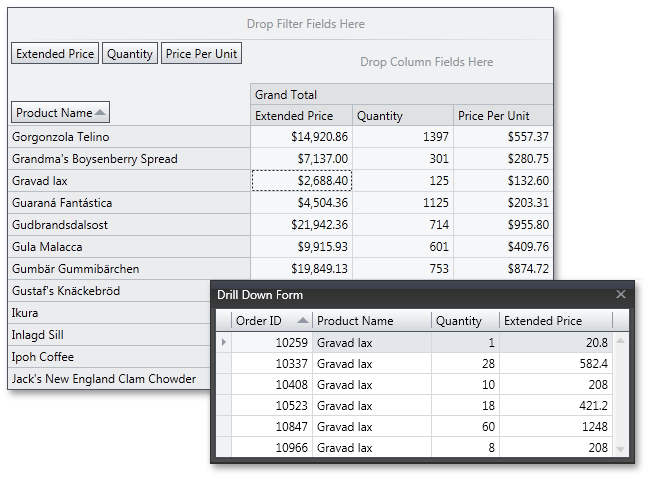# PivotGridControl.DataFieldUnboundExpressionMode Property

Gets or sets whether unbound expressions for data fields are calculated based on the data source records, or summary values. This is a dependency property.

Namespace: DevExpress.Xpf.PivotGrid

Assembly: DevExpress.Xpf.PivotGrid.v22.2.dll

NuGet Package: DevExpress.Wpf.PivotGrid

## Declaration

``public UnboundExpressionMode DataFieldUnboundExpressionMode { get; set; }``

## Property Value

Type Description
UnboundExpressionMode

One of the UnboundExpressionMode enumeration members, specifying how the unbound expressions for data fields are calculated.

Available values:

Name Description
Default

An unbound expression is calculated against each data source record, and then the resulting values are summarized.

UseSummaryValues

An unbound expression is calculated against summary values.

## Remarks

The DataFieldUnboundExpressionMode property specifies the calculation mode for evaluating the fields within an expression. An expression can be calculated against each data source record, summarizing the resulting values. Another option is to use the field’s summary value in an expression.

The image below shows the DXPivotGrid with the drill down form invoked. The ‘Price Per Unit’ column is unbound, and the expression is specified as [Extended Price] / [Quantity].If the DataFieldUnboundExpressionMode property is set to UnboundExpressionMode.Default, the unbound expression is calculated against each data source record. The resulting values are summarized. In the pivot grid shown above, the ‘Price Per Unit’ column value in the ‘Gravad lax’ row is calculated as follows:

Sum([Extended Price] / [Quantity]) = 20.8 / 1 + 582.4 / 28 + 208 / 10 + 421.2 / 18 + 1248 / 60 + 208 / 8 = 132.6

If the DataFieldUnboundExpressionMode property is set to UnboundExpressionMode.UseSummaryValues, the expression is calculated using summary values, as follows:

Sum([Extended Price]) / Sum([Quantity]) = (20.8 + 582.4 + 208 + 421.2 + 1248 + 208) / (1 + 28 + 10 + 18 + 60 + 8) = 21.5

Note

Unbound expressions evaluated against hidden fields and fields located in the Filter Area return the ‘Error’ value if the DataFieldUnboundExpressionMode property is set to DataFieldUnboundExpressionMode.UseSummaryValues, because summary values for these fields are not calculated.

Note

In the DataFieldUnboundExpressionMode.Default mode when an unbound expression is calculated against each data source record, you can refer to a field using the PivotGridFieldBase.FieldName property. If you enable the DataFieldUnboundExpressionMode.UseSummaryValues mode, use the PivotGridFieldBase.Name property instead. In both modes you can refer to a field using the PivotGridField.ExpressionFieldName property, which automatically returns the required identifier based on the currently enabled mode.

To specify an unbound expression mode for a single field, use the field’s PivotGridField.UnboundExpressionMode property.